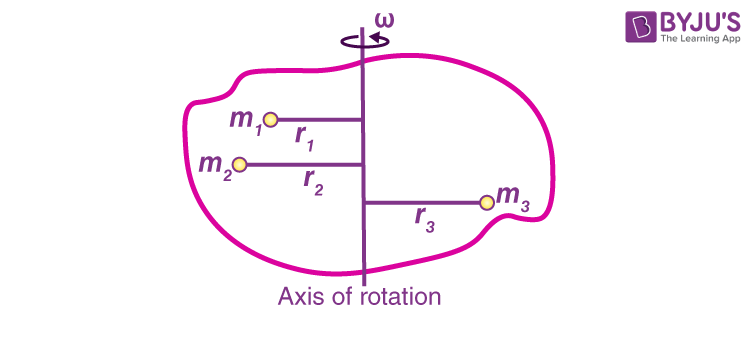# Derive an expression for torque in terms of the moment of inertia.

Solution:

Consider a rigid body rotating about a given axis with a uniform angular acceleration α, under the action of torque.

Let the body consist of particles of masses m1, m2, m3, . . … , mn at a distance r1, r2, r3, . . … rn respectively from the axis of rotationIf a1, a2, a3, . . … , an are the respective linear acceleration of the particles, then,

a1 = r1α, a2 = r2α, a3 = r3α…..

Force on particles of mass m is

f1 = m1a1 = m1r1α

Moment of this force about the axis of rotation f1 × r1 = (m1r1α) × r1 = m1 r12α

Similarly, the moment of forces on other particles about the axis of rotation is

m2 r22α, m3 r32α ———-mn rn2α

Torque acting on the body

τ = m1 r12α + m2 r22α + m3 r32α ———-mn rn2α

So,

$$\begin{array}{l}\vec{\tau }= \sum_{i=0}^{i=n}m_{i}r_{i}^{2}\alpha \end{array}$$

τ = Iα

where m1 r12 = moment of inertia of the body about the given axis of rotation(14)(4)